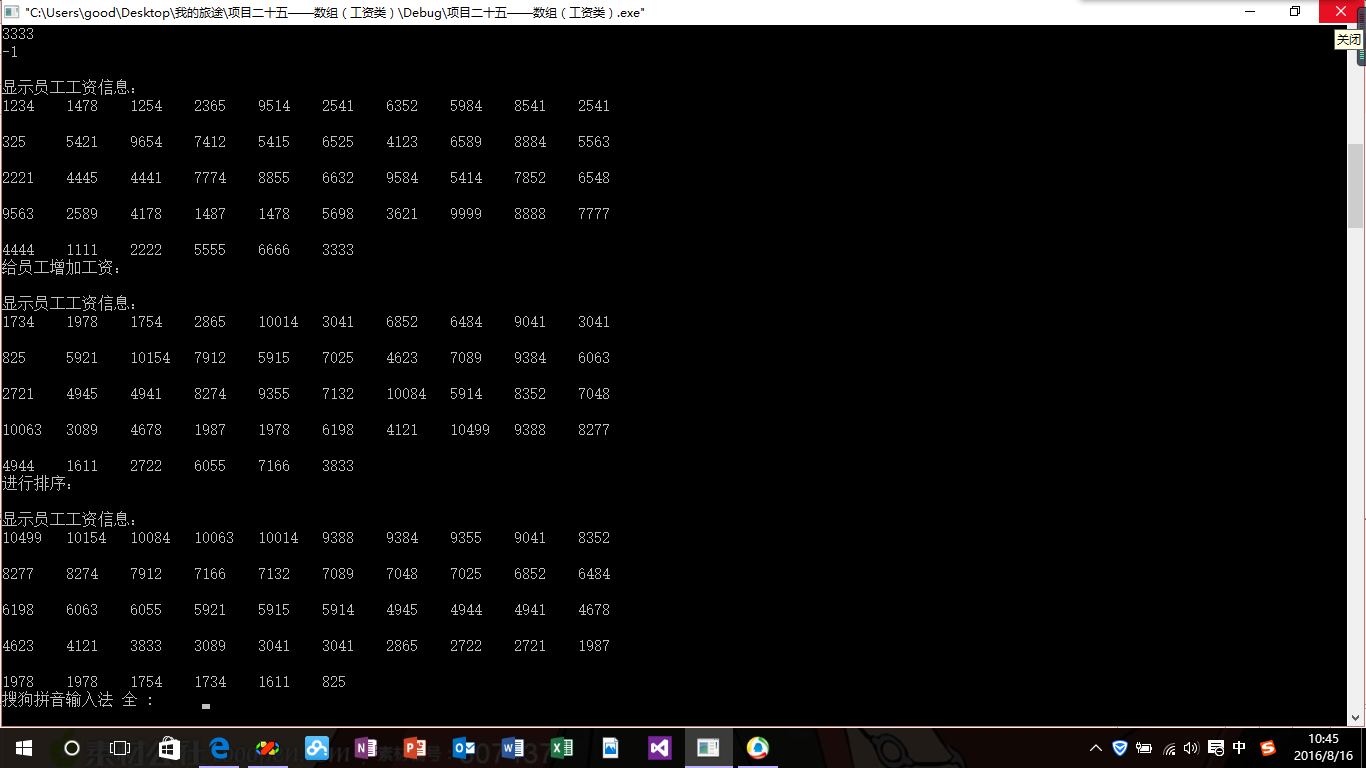工资类（数组）-手动输入

（1）实现Salary类中的成员函数，在main函数定义Salary类的对象，输入工资，再给每个人涨500元工资，排序后工资数据，然后输出结果。
（2）手工输入工资？！太让人不能忍受了。现给出包含了不足500个职工工资的文件salary.txt（下载），增加一个成员函数，用于从文件中读出数据，再增加一个成员函数，将排序后结果保存到一个文件中。编写main函数，测试你扩充的功能。
（3）用多文件的方式组织最后的程序。

（1）salary.h

#ifndef SALARY_INCLUDE
#define SALARY_INCLUDE
class Salary
{
public:
void set_salarys( );//输入职工工资（输入-1标志着工资输入结束），工资保存到salary数组中，实际人数保存到number中；
void sort_salarys(); //对工资由大到小排序
void show_salarys( ); //显示工资信息
private:
double salarys; //多人的工资
int number;  //实际人数
};
#endif

（2）salary,cpp

#include<iostream>
#include"salary.h"
using namespace std;

void Salary::set_salarys()
{
//	static int i;
int i;
number=0;
cout<<"输入员工工资信息："<<endl;
for(i=0;i<50;i++)
{
cin>>salarys[i];
if(salarys[i]==(-1))
break;
number++;
}
cout<<endl;
}
void Salary::show_salarys()
{
cout<<"显示员工工资信息："<<endl;
int i=0;
while(salarys[i]!=(-1))
{
cout<<salarys[i]<<'\t';
i++;
if(i%10==0)
cout<<endl;
}
cout<<endl;

}
{
cout<<"给员工增加工资："<<endl;
for(int i=0;i<number;i++)
{
salarys[i]+=x;
}
cout<<endl;
}

void Salary::sort_salarys()
{
cout<<"进行排序："<<endl;
int i,j;
for(i=0;i<number;i++)
{
for(j=i;j<50;j++)
{
if(salarys[i]<salarys[j])
{
double temp=salarys[i];
salarys[i]=salarys[j];
salarys[j]=temp;
}
}
}
cout<<endl;
}

（3）main.cpp

#include<iostream>
#include"salary.h"
using namespace std;

int main()
{
Salary p;
p.set_salarys();
p.show_salarys();
p.show_salarys();
p.sort_salarys();
p.show_salarys();
return 0;
}